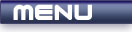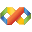Home Latest News Archive Tutorials C++ 2 3 4 Win32 Java OpenGL GLUT OpenGL ES 2 3 4 DirectX VB.NET HTML Photoshop 3dsmax Forums Projects Modelling Links About Us Contact Search Recent Tutorials C / C++ Win32 OpenGL OpenGL ES GLUT## Function Parameters

Tutorials > C++ > Function Parameters

### Introduction

Sometimes you may find that you need to pass information onto the function. This may determine the output of your function or the actions that are taken. You could make all your variables global and then reference them in your functions, but this makes your program harder to understand and it is not immediately apparent as to what variables you are currently working with.

Contents of main.cpp :

```#include <iostream>
#include <stdlib.h>

using namespace std;
```

Our function, printSequence takes 2 parameters. Each parameter is separated by commas. The parameters take the form similar to the declaration of a variable :

data type variable name

These can now be used as any other variable in your function. The function below takes a starting number and an ending number. With these numbers it prints each number from start to end.

```void printSequence(int start, int end)
{
for (int i = start; i <= end; i++)
cout << i << endl;
}
```

Our next example shows how return values work. The value being returned must be the same data type as was shown in the function declaration.
The function, sumNumbers also takes a start and end integer but this time the function keeps track of the sum of all these numbers in a variable called sum. This value is returned from the function.

```int sumNumbers(int start, int end)
{
int sum = 0;

for (int i = start; i <= end; i++)
sum += i;

return sum;
}
```

When the function is called, the extra parameters must now be given. This can be seen below where 5 and 10 are passed to the printSequence function.

```int main()
{
printSequence(5, 10);
```

The example below shows how a return value can be dealt with. You can see the function call as basically being replaced by its result. We therefore create a variable sum and make it equal to the sum of all numbers from 5 to 10.

```	int sum = sumNumbers(5, 10);

cout << "Sum : " << sum << endl;
```

It is not essential to assign the return value to a variable. As I said before, imagine that the function call is being replaced by the result. We can therefore place it in the middle of a statement. This can be seen below where the function call is placed as one of the outputs for the cout object.

```	cout << "Sum : " << sumNumbers(5, 10)
<< endl;

system("pause");

return 0;
}```

Congratulations. You should now be able to pass extra information to your functions via parameters.

Please let me know of any comments you may have : Contact Me

 Source Files :< Tutorial 20 - Functions Tutorial 22 - References >